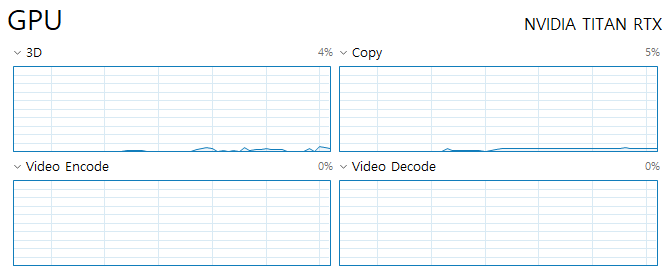# High CPU usage, How can I deal with CPU consumption?

Hello.
I have a question about CPU consumption.
When running U-net using pytorch, CPU usage is high.I’m dealing with a 512 * 512 medical image of 6720 slices, and the shape of the input numpy has [6720,512,512], file size has 1720322KB.
The project being executed is segmentation using U-Net model.

The GPU is using TITAN RTX, CPU is Intel i7-9700K and RAM is 32GB.

I created a custom dataset using ‘torch.utils.data.Dataset’, and the code is shown below.

``````class trainDataset(torch.utils.data.Dataset):
def __init__(self, data, target, augmentation=True):
self.data = data
self.target = target

self.augmentation = augmentation
def __getitem__(self, index):
x = self.data[index]
y = self.target[index]

x, y = self.transform(x, y)

return x, y

def transform(self, data, target):
data, target = data_augmentation(data, target, self.augmentation)
return data, target

def __len__(self):
return len(self.data)
``````

I thought that the data augmentation function in the custom dataset could consume a lot of CPU, so I ran it without applying it. However, the CPU consumption is still high.

Then, the model and train both input and target are calculated on the GPU as shown below. However, the CPU consumption is still high.

``````def fit(epoch,model,data_loader,phase='train',volatile=False):
if phase == 'train':
model.train()
if phase == 'valid':
model.eval()

running_loss = 0.0
for batch_idx , (data,target) in enumerate(data_loader):
inputs,target = data.to(device),target.to(device)

output = model(inputs)
loss = criterion(output,target.long())

if phase == 'train':
loss.backward()
optimizer.step()

running_loss += loss.data.item()

if phase == 'train':
exp_lr_scheduler.step()

print('{} Loss: {:.4f}'.format(
phase, loss))
return loss
``````
``````for epoch in range(num_epochs):
print()
print('Epoch {}/{}'.format(epoch, num_epochs - 1))
print('-' * 10)